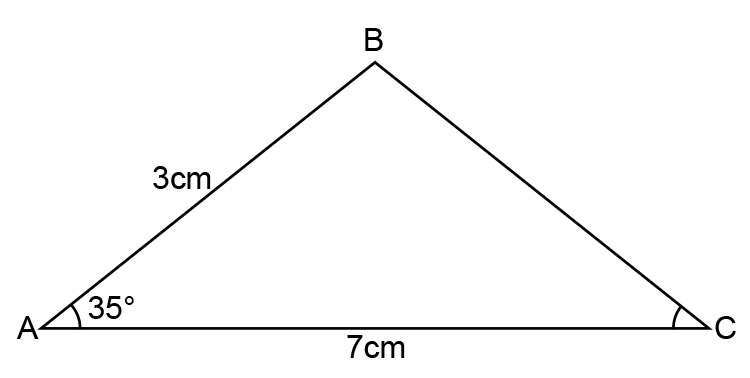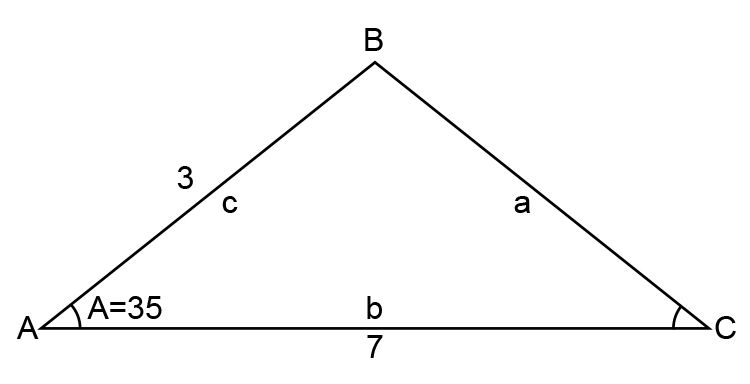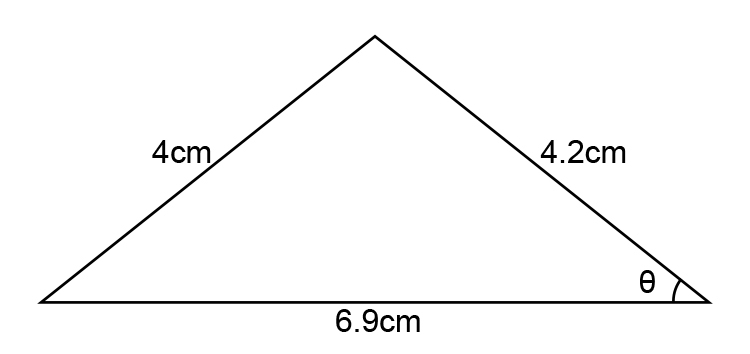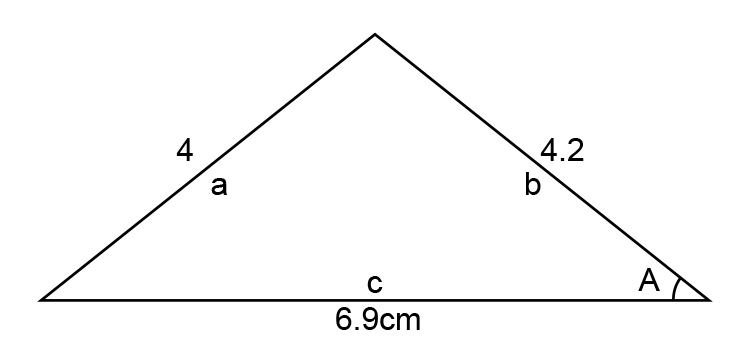# Examples using cosine rule

Example 1

You can use the cosine rule (If you can remember it – but why try) here it is anyway:

What is the length B to C in the following diagram?Cosine rule is:    a^2=b^2+c^2-2bc\ cosA

Redraw the diagramTherefore  a^2=b^2+c^2-2bc\ cosA

Becomes:   a^2=7^2+3^2-2times7times3cos35^@

a^2=49+8-42cos35^@

a^2=58-42cos35^@

a^2=58-34.4

a^2=23.6

a=sqrt 23.6

a=4.85cm

Example 2

In the diagram below find thetaCosine rule is:    a^2=b^2+c^2-2bc\ cosA

Redraw the diagramRearrange the cosine rule:

-cosA=(a^2-b^2-c^2)/(2bc)

-cosA=(4^2-4.2^2-6.9^2)/(2times4.2times6.9)

-cosA=(16-17.64-47.61)/57.96

-cosA=(-49.25)/57.96

Swap sides to cancel out the negative

49.25/57.96=cosA

0.849=cosA

Answer: A=31.89^@